MPAE-W-100-94-27

MAX-PLANCK-INSTITUT F‹R AERONOMIE
D-37191 Katlenburg-Lindau, Federal Republic of Germany ______________

The Tetrahedron Quality Factors of CSDS
 PATRICK W. DALY
1994 June 7
This report available from
`http://www.mpae.gwdg.de/~daly/tetra.html`

 Abstract
 The four Cluster spacecraft will form a tetrahedron, which ideally should be a regular one: equal spacing between all pairs of vertices. In reality, this will not be the case. A number of parameters exist to specify how badly off the true figure is. This paper presents some mathematics of tetrahedrons, describes the two parameters that are to be used in the Cluster Science Data System, and gives a Fortran program for their calculation.

## Introduction†

Four points in space define a tetrahedron. If the separations between each pair of points are equal, then it is a regular tetrahedron. The four Cluster spacecraft will form a tetrahedron, which in general is not regular. How can we specify the degree to which regularity is achieved?

### The "Glassmeier" Parameter†

 Table†1 Special values of the Glassmeier parameter
 QG Meaning 1.0 The four points are colinear 2.0 The points all lie in a plane 3.0 A regular tetrahedron is formed

The parameter proposed by vom Stein, Glassmeier, and Dunlop†(1992) is defined as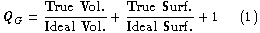†
and takes on values between 1 and 3. It tends to describe the dimensionality of the figure, as listed in Table†1. The ideal volume and surface are calculated for a regular tetrahedron with a side length equal to the average of the 6 distances between the 4 points.

### The "Robert/Roux" Parameter†

In their paper on tetrahedron shape, Robert and Roux†(1993) present 17 different parameters, as ratios of various volumes, sizes, areas. Of these, the CSDS community has decided to adopt one as its second quality parameter for the auxiliary data. It is defined as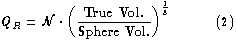†
where the sphere is that circumscribing the tetrahedron (all four points on its surface) and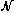is a normalization factor to make QR = 1 for a regular tetrahedron. The range of values is between 0 and 1.

## Mathematics of a Tetrahedron†

Consider four points in space and the figure formed by joining them with lines (Figure†1). The points are numbered 0 to 3, and their vectors are r 0, r1, r2, r3. Without any loss of generality, we may consider only the differences

`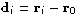`

in describing the points.Figure†1 A tetrahedron and its four vertices.

### Area of a Side†

The area of a parallelogram bounded by two vectors d1 and d2 is given by the magnitude of their cross product; any triangle is half of a parallelogram, so its area is

`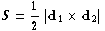`

where d1 and d2 are the vectors for any two sides of the triangle (Figure†2).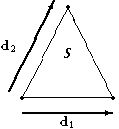Figure†2 Area S of a triangle.

For the four sides of the tetrahedron, specify side n to be that one that does not contain point n at any of its vertices. Thus:

 S1 =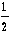|d2 ◊ d3| (3) S2 =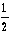|d1 ◊ d3| (4) S3 =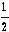|d1 ◊ d2| (5) S0 =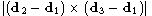=|d1◊d2 + d2◊d3 + d3◊d1| (6)
The total surface S is the sum Ś n=03Sn.

### Volume of a Tetrahedron†

The volume of a figure bounded by three vectors in space is the triple product of those vectors. Any tetrahedron is 1/6 of such a figure, hence
 V =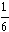|d1 ∑ d2 ◊ d3| (7) =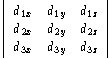(8)

### Center of Circumscribed Sphere†

To find the circumscribed sphere, we need the point that is equidistant from all four vertices, i.e. we want r such that
 (r - rn) ∑ (r - rn) = r2; "n = 0, 3 r2 - 2r ∑ r n + rn2 = r2
If we take point 0 as the origin, that is, if we use the dn vectors in place of the rn, then r2 = r2, the sphere radius, and the above 4 equations reduce to

`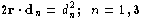`

This yields the matrix equation for the center of the sphere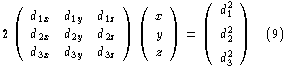†
which can be solved for the vector (x, y, z) and the radius of the sphere r2 = x2 + y2 + z2. Note that the leftmost matrix in equation†9 is the same as the one whose determinant yields the volume of the tetrahedron (equation†8).

The volume of the circumscribed sphere is then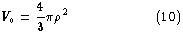†

## The Regular Tetrahedron†

The regular tetrahedron of unit side is the ideal against which the true figure of the four spacecraft is to be measured. We may take
 d0 = (0, 0, 0) d1 = (1, 0, 0) d2 =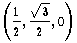d3 =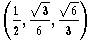Table†2 Values for regular tetrahedron
 Quantity Value S0 †=†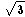/4 S †=†V †=†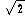/12 r †=†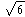/4 V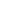†=†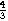p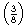†=†

Values for the regular tetrahedron of unit side length are listed in Table†2.

## Calculating the Quality Factors†

The quality factors in equations†1 and 2 can now be found with the help of these formulas.

For QG, we average the 6 distances between the 4 points to get the side L of the "ideal" regular tetrahedron, with volume L3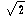/12 and surface L2. The true volume and surface are found from equations†7 and 3-6.

For QR, the radius of the circumscribing sphere is calculated from equation†9. The actual volume of the sphere need not be calculated, for all the factors just go into the normalizing.

`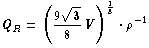`

A Fortran program calculates both these parameters.

## References†

 †† Robert, P. and Roux, A. (1993). Influence of the Shape of the Tetrahedron on the Accuracy of the Estimate of the Current Density. Proceedings of ESA `START' Conference, Aussois, France, Centre de Recherches en Physique de l'Environment, Issy les Moulineaux, France. †† vom Stein, R., Glassmeier, K.-H., and Dunlop, M. (1992). A Configuration Parameter for the Cluster Satellites. Tech.†Rep.†2/1992, Institut fŁr Geophysik und Meteologie der Technischen Universitšt Braunschweig.